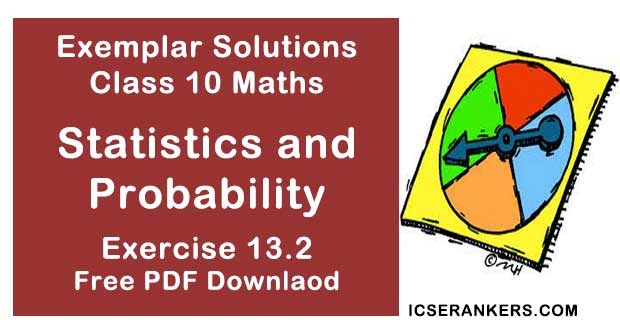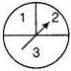# Chapter 13 Statistics and Probability NCERT Exemplar Solutions Exercise 13.2 Class 10 MathsChapter Name NCERT Maths Exemplar Solutions for Chapter 13 Statistics and Probability Exercise 13.2 Book Name NCERT Exemplar for Class 10 Maths Other Exercises Exercise 13.1Exercise 13.3Exercise 13.4 Related Study NCERT Solutions for Class 10 Maths

### Exercise 13.1 Solutions

1. The median of an ungrouped data and the median calculated when the same data is grouped are always the same. Do you think that this is a correct statement? Give reason.

Solution

Not always, because for calculating median of a grouped data, the formula used is based on the assumption that the observations in the classes are uniformly distributed (or equally spaced).

2. In calculating the mean of grouped data, grouped in classes of equal width, we may use the formula, x = a + (Σfi di )/(Σfi ) Where, a is the assumed mean, a must be one of the mid-point of the classes. Is the last statement correct? Justify your answer.

Solution

No, it is not necessary that assumed mean consider as the mid-point of the class interval. It is considered as any value which is easy to simplify it.

3. Is it true to say that the mean, mode and median of grouped data will always be different? Justify your answer.

Solution

The value of these three measures can be the same, it depends on the type of data.

4. Will the median class and modal class of grouped data always be different? Justify your answer.

Solution

Not always, It depends on the given data.

5. In a family having three children, there may be no girl, one girl, two girls or three girls. So, the probability of each is 1/4 . Is this correct ? Justify your answer.

Solution

No, the probability of each is not  1/4 because the probability of no girl in three children is zero and probability of three girls in three children is one.

Justification
So, these events are not equally likely as outcome one girl, means gbb, bgb, bbg ‘three girls’ means ‘ggg’ and so on.

6. A game consists of spinning an arrow which comes to rest pointing at one of the regions (1, 2 or 3) (see figure). Are the outcomes 1, 2 and 3 equally likely to occur? Give reasonsSolution

No, the outcomes are not equally likely, because 3 contains half part of the total region, so it is more likely than 1 and 2, since 1 and 2, each contains half part of the remaining part of the region.

7. Apoorv throws two dice once and computes the product of the numbers appearing on the dice. Peehu throws one die and squares the number that appears on it. Who has the better chance of getting the number 36? Why?

Solution

Apoorv throws two dice once.
So total number of outcomes = 36
Number of outcomes for getting product 36 = 1 (6 ×  6)
∴ Probability for Apporv = 1/36
Also, Peehu throws one die,
So, total number of outcomes = 6
Number of outcomes for getting square 36 = 1(62 = 36)
∴ Probability for Peehu = 1/6 = 6/36
Hence, Peehu has better chance of getting the number 36.

8.When we toss a coin, there are two possible outcomes-head or tail. Therefore, the probability of each outcome is 1/2. Justify your answer.

Solution

Yes, probability of each outcome is 1/2 because head and tail both are equally likely events.

9. A student says that, if you throw a die, it will show up 1 or not 1. Therefore, the probability of getting 1 and the probability of getting not 1. each is equal to 1/2. Is this correct? Give reasons.

Solution

No, this is not correct.
Suppose we throw a die, then total number of outcomes = 6
Possible outcomes = 1 or 2 or 3 or 4 or 5 or 6
∴ Probability of getting  1 = 1/6
Now, probability of getting not 1 = 1 - Probability of getting 1
= 1 - 1/6 = 5/6

10. I toss three coins together. The possible outcomes are no heads, 1 head,2 head and 3 heads. So, I say that probability of no heads is 1/4. What is wrong with this conclusion?

Solution

I toss three coins together  [given]
So, total number of outcomes = 23 = 8
and possible outcomes are (HHH), (HTT), (THT), (TTH),(HHT), (THH), (HIH)and (TTT)
Now, probability of getting no head = 1/8
Hence, the given conclusion is wrong because the probability of no head is 1/8 not  1/4

11. If you toss a coin 6 times and it comes down heads on each occasion. Can you say that the probability of getting a head is 1? Given reasons.

Solution

No. if let we toss a coin, then we get head or tail, both are equally likely events So, probability is 1/2. If we toss a coin 6 times, then probability will be same in each case. So, the 2 probability of getting a head is not 1.

12. Sushma tosses a coin 3 times and gets tail each time. Do you think that the outcome of next toss will be a tail? Give reasons.

Solution

The outcome of next toss may or may not be tail, because on tossing a coin, we get head or tail so both are equally likely events.

13. If I toss a coin 3 times and get head each time, should I expect a tail to have a higher chance in the 4th toss? Give reason in support of your answer.

Solution

No, let we toss a coin, then we get head or tail, both are equaly likely events i.e., probability of each event is 1/2. So, no question of expecting a tail to have a higher chance in 4th toss.

14. A bag contains slips numbered from 1 to 100. If Fatima chooses a slip at random from the bag, it will either be an odd number or an even number. Since, this situation has only two possible outcomes, so the 1 probability of each is 1/2. Justify.

Solution

We know that, between 1 to 100 half numbers are even and half numbers are odd i.e., 50 numbers (2, 4, 6, 8.... 96, 98,100) are even and 50 numbers (1,3, 5, 7. . , 97, 99) are odd. So, both events are equally likely.
So, probability of getting even number = 50/100 = 1/2
and probability of getting odd number = 50/100  = 1/2
Hence, the probability of each is  1/2.